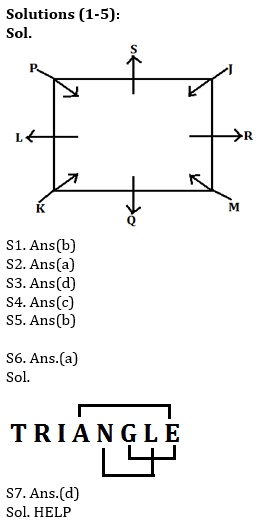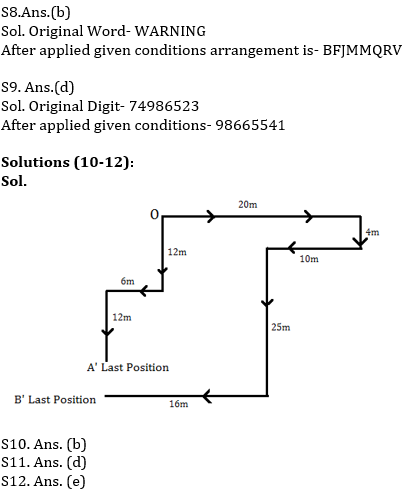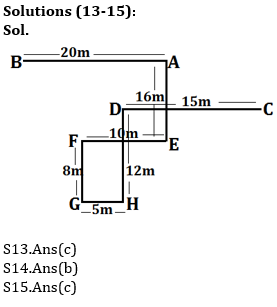Latest Banking jobs   »   Reasoning Ability Quiz For IBPS RRB...

# Reasoning Ability Quiz For IBPS RRB PO, Clerk Prelims 2021- 11th August

Directions (1-5): Study the following information carefully and answer the questions given below:
Eight persons J, K, L, M, P, Q, R and S are the sitting around the square table in such a way four of them sit at four corners and face the center while four of them sit in the middle of each of the four sides and face outside.
J faces the center and sits third to the left of Q. P faces the center and is not an immediate neighbour of Q. Only one person sits between Q and R. M sits second to right of K, who faces the center. L is not an immediate neighbour of J.

Q1. Who among the following are immediate neighbours of S?
(a) R, M
(b) P, J
(c) P, L
(d) J, M
(e) K, M

Q2. What is the position of L with respect to P?
(a) Immediate right
(b) Second to the right
(c) Immediate left
(d) Third to the left
(e) None of these

Q3. Four of the following five belong to a group in a certain way, find which of the one does not belong to the group?
(a) S
(b) L
(c) R
(d) M
(e) Q

Q4. Who among the following person sits opposite to R?
(a) S
(b) M
(c) L
(d) K
(e) None of these

Q5. How many maximum persons can sit between P and M?
(a) 6
(b) 3
(c) 2
(d) 4
(e) 5

Q6. How many pairs of letters are there in the word ‘TRIANGLE’, each of which have as many letters between then in the word as they have between then in the English alphabet (Both Forward and Backward direction)?
(a) Three
(b) One
(c) Two
(d) More than Three
(e) None

Q7. How many words can be formed from the 1st, 4th, 7th and 8th letter of a word ‘PUBLISHED’ by using each letter once in the word?
(a) More than Three
(b) Three
(c) Two
(d) One
(e) None

Q8. If in the letter ‘WARNINGS’ vowels are replaced with its next letter and consonant is replaced with its previous letter. After that all letters are arranged in alphabetical order, then which of the following letter is fourth from the right end?
(a) R
(b) M
(c) B
(d) F
(e) Q

Q9. If in the number 74986523, 1 is added to second, fourth, sixth and eighth digit and 1 is subtracted from the first, third, fifth and seventh digits, then all the numbers are arranged in descending order from left to right. Which of the following is fifth digit from left end in the new arrangement?
(a) 4
(b) 8
(c) 6
(d) 5
(e) None of these

Directions (10-12): Read all the information carefully and answers the following questions given below:
Point O is the Initial Point of A and B. From point O, A Goes 12m south direction and takes his right and walks 6m. Now he takes his left and walks 12m to reach his destination. From point O, B goes 20m in east direction and takes his right and walks 4m and takes another right turn and walks 10m. Now he takes his left and walks 25m after that he takes right turn and goes 16m to reach his final destination.

Q10. Find the total distance travel by B?
(a) 55m
(b) 75m
(c) 45m
(d) 95m
(e) 65m

Q11. What is the position of Point O with respect to A’s last position?
(a) North-West
(b) North
(c) South-East
(d) North-East
(e) None of these

Q12. What is the difference between total distance travel by A and total distance travel by B?
(a) 30m
(b) 35m
(c) 40m
(d) 42m
(e) None of these

Directions (13-15): Study the information carefully and answer the questions given below.
Point G is 5m west of point H, which is 12m south of point D. Point A is 20m east of point B. Point F is 10m west of point E. Point C is 15m east of point D. Point F is 8m north of point G. Point E is 16m south of point A.

Q13. What is the direction of point G with respect to point A?
(a) South-east
(b) North-west
(c) South-west
(d) West
(e) None of these

Q14. What is the shortest distance between point H and point E?
(a) 8m
(b) √89m
(c) 10
(d) √15m
(e) None of these

Q15. What is the direction of point B with respect to point C?
(a) East
(b) North
(c) North-west
(d) South-East
(e) None of these

Solutions